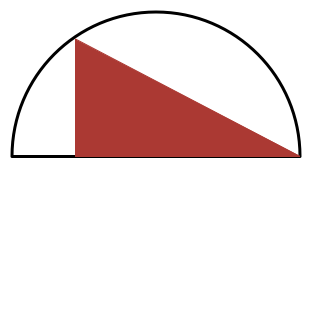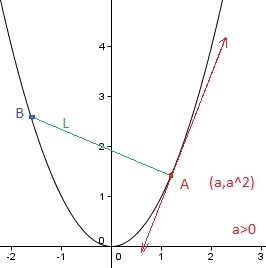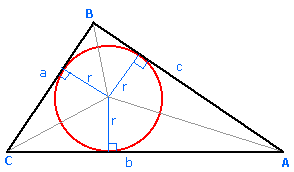Calculus

# Extrema: Level 3 ChallengesA circle rests in the interior of the parabola with equation $y=x^2$ so that it is tangent to the parabola at two points. How much higher is the center of the circle than the points of tangency?

Source: Mandelbrot #2Let $S$ be the maximum possible area of a right triangle that can be drawn in a semi-circle of radius $1$, where one of the legs (and not the hypotenuse) of the triangle must lie on the diameter of the semicircle.

If $S = \dfrac{a\sqrt{b}}{c},$ where $a,c$ are positive coprime integers and $b$ is a positive square-free integer, find $a + b + c.$

How many real values of $x$ satisfy the equation

$\large {x}^{2}-{2}^{x}=0?$Suppose that two people, A and B, walk along the parabola $y=x^2$ in such a way that the line segment $L$ between them is always perpendicular to the line tangent to the parabola at A's position $(a,a^2)$ with $a > 0$. If B's position is $(b,b^2)$, what value of $b$ minimizes $L$?What is the $least$ $perimeter$ of an isosceles triangle in which a circle of radius $\sqrt{3}$ can be inscribed ?

Note : The picture shown is a rough one.

×